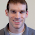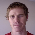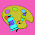## Thursday, March 17, 2011

### Computing phylogenetic signal

I have posted a new function to compute phylogenetic signal for continuous traits using two methods: the lambda method of Pagel (1999) and Blomberg et al. (2003)'s K-statistic. Rich Glor already created a very helpful wiki page on how to do this in R using several different functions. My function, phylosig() (code here), should make this even easier. With phylosig() you can specify method (presently either "K" or "lambda") and optionally return a P-value for either the randomization test of Blomberg et al. or a likelihood ratio test against the null hypothesis that lambda=0.

For instance, after loading our function from source, to compute K and conduct a hypothesis test of the null hypothesis of no signal we just type:

> phylosig(tree,x,test=TRUE)
\$K
 1.053583

\$P
 0.001

By default, the function uses method="K" and nsim=1000.

Next, we can also estimate lambda using maximum likelihood:

> phylosig(tree,x,method="lambda",test=TRUE)
\$lambda
 0.9999339

\$logL
 -132.7309

\$P
 0

Now, for comparison, let's simulate data with no phylogenetic signal:

> x<-rnorm(100); names(x)<-tree\$tip.label
> phylosig(tree,x,test=TRUE)
\$K
 0.1020861

\$P
 0.426

> phylosig(tree,x,method="lambda",test=TRUE)
\$lambda
 6.610696e-05

\$logL
 -138.0889

\$P
 1

Cool. Please check out the function (on my R-phylogenetics page) and let me know how it works.

#### 34 comments:

1.Hi Liam,

Nice function. It seems faste than K estimation by phylosignal by a bit (esp at large numbers of iterations), and MUCH MUCH faster than geiger's lambda estimation.

I had a question: Do you know the relative strengths of K vs. lambda relative to tree size? I know a paper a few years back suggested that trees with ~20 species are too small to estimate K robustly, but what about lambda?

Thanks, Scott

2.Liam-
I'll try it out on my big graptolite supertree and let you know how it goes. If its much faster than geiger's lambda estimation, like Scott says, than I'm already a happy man.

Scott-
Do you have a reference for that paper that talked about trees being too small to get a good estimate of K?

Thanks,
-Dave

3.Dave,

Blomberg et al. 2003 Evolution is where I have seen power in relation to tree size.

Scott

4.@Scott - you could try investigating this by simulating and looking at the variance in K across simulations. Just an idea.

@Dave - please let me know about your success (or failure) using this or any of my other functions with especially large phylogenies. I have not tested these functions, in most cases, for more than a few hundred species.

5.Liam, thanks for the post. But I haven't been able to get it to work. It stops at one of the first error messages:

Error in phylosig(tree, y, method = "lambda") :
some species in tree are missing from data.

Any suggestions?

6.Hi Nicole.
Are some species in the tree missing from the data? (Note that the data should be a vector with names equal to the species names.)
To check, you can try the name.check() function in the "geiger" package. This function, I believe, returns a list containing two elements - the species found in the data, but not the tree; and the species found in the tree but not the data.
I hope this is of some help.
- Liam

7.Data and tree have the same number of taxa and in the same order...

-Nicole

8.Hi Nicole. The data vector for your continuous trait, x, still has to have names. If your data vector is in the same order as tree\$tip.label, then you can just do:

> names(x)<-tree\$tip.label

Of course, if the order of x is actually *different* from the order in \$tip.label, then protocol this will just enable you get an erroneous result (rather than no result at all), so be very careful!

9.Hi, Can Phylosig() handle missing data (i.e. entered as NA) in the traits? Since the phylogeny i am using has many more species than i have data for.
-Christofer

10.This comment has been removed by the author.

11.Hi Christofer.

The present version cannot handle missing data (entered as NA or absent from the data array), but I am working on a version that can - and I will upload it shortly.

You are correct phylosig() - and, I believe, BM model based methods for calculating phylogenetic signal generally - cannot handle 0 length terminal edges.

12.Done. See my blog post here or v0.2 code here.

13.This works great! I entered a data set with 65 species, then read in a nexus file with 76 species (i did not add lines with NA for the extra 11 species). The analysis worked and i confirmed the result by doing the calculations from the distance matrix. I also assume the code re-orders that data after it removes taxa from the tree (which i think is this code - tree<-drop.tip(tree,tree\$tip.label[-match(names(x),tree\$tip.label)]).
I also had trouble with my analysis as i had branch lengths of 0 at the tip, but i overcame this by replacing them with very small numbers (i.e 0.000001).
Anyway great code, thanks Liam!

14.Christofer.
Actually, that code drops the tips of the tree that are not in x.

Once we have the same set of species in x & the tree, reordering x is simple:
C<-vcv.phylo(tree); x<-x[rownames(C)]

I'm glad the code worked for you!

15.Hi all,

Here is a simulation I did to examine how K and lambda vary in response to tree size.

Two observations: First, it seems that lambda is more sensitive than K to tree size, but then lambda levels out at about 40 species, whereas K continues to vary around a mean of 1.

Second, K is more variable than lambda at all levels of tree size (compare standard error bars).

Here is the output figure: https://picasaweb.google.com/lh/photo/d38ZwL5bsZMt_2jSxvNkMA?feat=directlink

I am curious if I did anything wrong below, if it makes sense, etc.

#### Simulations
install.packages(c("ape","reshape2","ggplot2"))
require(ape); require(reshape2); require(ggplot2)
source("http://anolis.oeb.harvard.edu/~liam/R-phylogenetics/phylosig/v0.3/phylosig.R")
source("http://anolis.oeb.harvard.edu/~liam/R-phylogenetics/fastBM/v0.4/fastBM.R")

# Simulation function physig_sim
# input: x = number of species in tree
# output: a vector length two with (K, lamba)
physig_sim <- function(x) {
tree <- rcoal(x)
traits <- fastBM(tree)
physig_k <- phylosig(tree, traits, method = "K")
physig_l <- phylosig(tree, traits, method = "lambda")\$lambda
sigs <- c(physig_k, physig_l)
return(sigs)
}

# Run simulation
spnumbs <- c(5, 10, 15, 20, 25, 30, 35, 40, 45, 50, 65, 80, 95)
rands <- 1000
siglist <- alply( spnumbs, 1, function(x) t(replicate(rands, physig_sim(x))), .progress="text")
sigdf <- as.data.frame(do.call(rbind, siglist))
names(sigdf) <- c("K", "L")
sigdf\$numsp_ <- rep(spnumbs, each=rands)
sigdf_m <- melt(sigdf, id = 3)

# Plot results
plotdf <- ddply(sigdf_m, .(numsp_, variable), summarise,
mean = mean(value),
se = sd(value)/sqrt(length(value))
)
limits <- aes(ymax = mean + se, ymin = mean - se)
dodge <- position_dodge(width=0.9)
ggplot(plotdf, aes(x = numsp_, y = mean, shape = variable)) +
geom_point(position=dodge) +
geom_errorbar(limits, position=dodge, width=0.25) +
geom_smooth()
ggsave("physig_sim.jpeg")

16.Is there a code for a phylogenetic signal test for discrete unordered characters? I am looking for something similar to K and lambda but for discrete characters
Thanks in advance

Julissa Roncal

17.Hi Liam,

This function is amazingly faster than geiger!!! I am having a small problem I can get everything to work for the K stats no problems but when I try to simulate no phylogenetic signal for lambda i get this error message "Error in invCl %*% x : non-conformable arguments". I was hoping you might have some useful suggestion.

Thanks in advance

Vanessa

18.Hi Vanessa.

Thanks for your comment.

I'd love to help, but I need more details in order to try and reproduce your error. Can you either post the code that you used to produce the error or send me the data via email (liam.revell@umb.edu)?

Thanks again. - Liam

19.Hi Liam,

Please, in which line do I put my tree and traits? For the simulation comparing K and Lambda.

Many thanks!

20.Hi - to run phylosig(), you first need to read in a vector of your data and your tree as a "phylo" object (created using, for instance, read.tree). Then you just type:

result<-phylosig(tree,x,method="lambda") # for instance.

The vector, x, should have names (in names(x)) that correspond with the tip labels in your tree (contained in tree\$tip.label).

I hope this is of some help.

All the best, Liam

21.Hi Liam,
I was actually referring to the physig_sim that compares the response of K or Lambda on tree size. I want to know which is best for my data of over 200 species. I have tried to call the function by: physig_sim(tree, trait), but it didn't work?

Also, it may probably be better to say phylosig() doesn't estimate multiple traits at the same time.

Many Thanks!

Charlotte

22.Hi Charlotte.

physig_sim is by Scott Chamberlain (here) not myself, although it looks like he calls phylosig from the phytools package.

If you want to do joint estimation of lambda for multiple traits you could use phyl.pca in phytools, which optionally does this.

- Liam

23.@Charlotte: Let me know if you want help altering the physig_sim function.

Scott

24.This just made my day. I've been trying to figure out why the fitContinuous examples under "Phylogenetic Signal" compare lnl under a lambda vs. BM model rather than under lambda=0 vs lambda=observed. And today I also ran into the arbitrary upper limit on beta you explained on R-sig-phylo. Not a problem here. So thanks very much, this is so much simpler.

25.Hi Liam,
Which reference can I use to refer to your function? Did you publish this somewhere?
Thanks,

1.Sure. The phytools package is described in a Methods in Ecology and Evolution publication, here.

26.Heather KharoubaJuly 26, 2012 at 5:08 PM

Hi Liam,

I'm probably missing something really simple here but can't figure it out. When I type:
phylosig(prunedTree,range,method="lambda",test=TRUE)

I get:
\$lambda
 0.9999339

\$logL
 -Inf

\$P
 NaN

Warning messages:
1: In optimize(f = likelihood, interval = c(0, 1), y = x, ... :
NA/Inf replaced by maximum positive value

Range varies from 25313 7561228 with no missing values. When I run other traits with the same tree, I don't get an error. Is my lambda that high or is there a problem with the trait?

Thanks,
Heather

27.Hi Heather.

Are you using the latest version of phytools? Or have you loaded this function from source.

Try installing/updating phytools and then re-run your analyses. If the error repeats, please let me know.

BTW, I don't have a good explanation as to why you would get this error even with old versions of phylosig/phytools, but it is more difficult for me to error check if you are not using the current version. (As well as less useful for other readers.)

Thanks so much for using phytools and reporting this issue.

All the best, Liam

1.Heather KharoubaJuly 26, 2012 at 7:10 PM

Hi Liam,

Originally, I had used phylosig from source. When I reload phytools, I don't get the error anymore. Thanks for the suggestion!

Thanks,
Heather

28.Great! - Liam

29.Hi Liam,

Great function to test phylogenetic signal. Thanks!
Can I ask if you know of any function (maybe implemented by your package) which deals with dynamic processes that change the tree?

For instance you have two trees and a specific sets of traits for each. How can I test if there is a specific trait driving the changes in the tree structure?

Many thanks again!
Inigo

30.Hi Liam,
I need to test the phylogenetic signal in morphological traits of several rodent species. The traits are: incisor procumbency, basilar length, mandibular width, out-lever arm and in-lever arms of jaw adductor, and mechanical advantages (in-lever arm divided by out-lever arm). I've tested phylogenetic signal using phylosig, one variable at a time, and i used raw data. But i'm not really sure if this is correct. Can you give me some advise?

Thanks for your time!
Alejandra

31.Hi Liam,

I have a question related to this topic. Is it necessary measure the phylogenetic signal of any variable in a ultrametric tree or it could be measure directly from a 16S rRNA tree?

Thanks,

Hilbert

32.Hi Liam

I have a question regarding the use and analysis of generated data. I have a tree from a NEXUS file and also my single trait in a csv file. How does one format these files in R to conduct the analysis using phylogsig?

Thanks!

Note: due to the very large amount of spam, all comments are now automatically submitted for moderation.